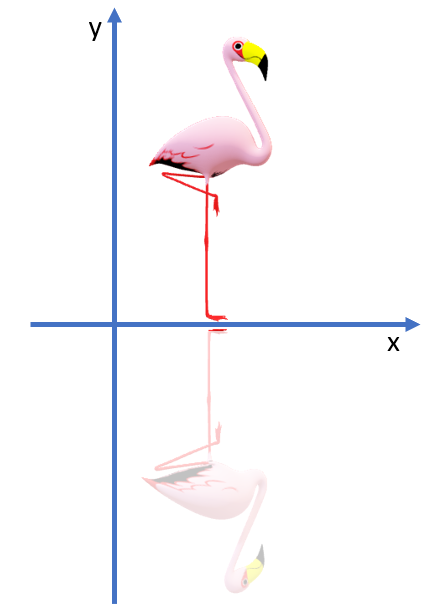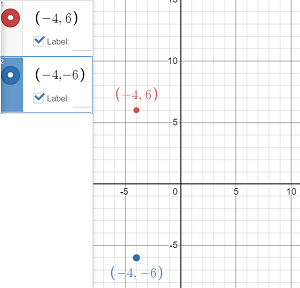# Reflection Over The X-AxisReflection over the x-axis is a type of linear transformation that flips a shape or graph over the x-axis. Every point above the x-axis is reflected to its corresponding position below the x-axis; Every point below the x-axis is reflected to its corresponding position above the x-axis.

Contents:
Reflection over the x-axis for:

## 1. Reflection Over The X-Axis: Sets of Coordinates

If you have a set of coordinates, place a negative sign in front of the value of each y-value, but leave the y-value the same.

Example question #1: Reflect the following set of coordinates over the x-axis:
(-4, 6), (-2, 4), (0, 0), (2, 4), (4, 6).

Solution:

(-4, -6), (-2, -4), (0, 0), (2, -4), (4, -6).

Step 2: (Optional) Plot both sets of coordinates (your original points and the ones from Step 2) to make sure you negated correctly. You can easily do this on Desmos.com: Just enter coordinates into the left hand column and check the “Label” box:## Reflecting Over The X-Axis: Functions

To reflect a function over the x-axis, multiply it by negative 1 (usually just written as “-“).

More formally:

When a function f(x) is reflected over the x-axis, it becomes a new function g(x) = – f (x).

Example Question #2: What is f(x) = x2 – 3 reflected over the x-axis?

Solution:
Step 1: Place a negative sign in front of the right-hand side of the function:
f(x) = x2 – 3 becomes g(x) = – (x2 – 3)

Step 2: Remove the parentheses, carrying through the negative sign:
g(x) = -x2 + 3.

Step 3: (Optional) Check your work by graphing both functions (your original function from the question and the one from Step 2) to make sure they are perfect reflections (I used Desmos.com):Note: I’m using f(x) and g(x) here to name the functions, but you can name them anything you like (or use whatever names your instructor is using). The important part of the formula is the expression on the right hand side.

## Matrix Operation for Reflection Over The X-AxisIn the Cartesian plane, a 2 x 2 matrix can describe a transformation on the plane. The above matrix A reflects a point (defined by column vector x) over the x-axis . For example, let’s say you had a point (1, 3) and wanted to reflect it over the x-axis. The matrix operation would be:## References

 Joyce, D. Some Linear Transformations on ℝ2. Math 130 Linear Algebra. Retrieved April 17, 2021 from: https://mathcs.clarku.edu/~ma130/lintrans2.pdf

CITE THIS AS:
Stephanie Glen. "Reflection Over The X-Axis" From StatisticsHowTo.com: Elementary Statistics for the rest of us! https://www.statisticshowto.com/reflection-over-the-x-axis/